Next: Charge Sheets and Dipole Up: Electrostatic Fields Previous: Electrostatic Energy

# Electric Dipoles

Consider a charge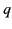located at position vector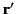, and a charge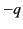located at position vector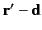. In the limit that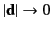, but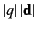remains finite, this combination of charges constitutes an electric dipole, of dipole moment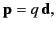(195)

located at position vector. We have seen that the electric field generated at point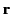by an electric chargelocated at pointis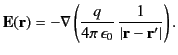(196)

Hence, the electric field generated at pointby an electric dipole of moment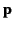located at pointis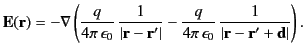(197)

However, in the limit that,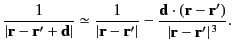(198)

Thus, the electric field due to the dipole becomes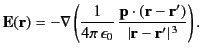(199)

It follows from Equation (154) that the scalar electric potential due to the dipole is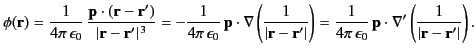(200)

(Here,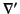is a gradient operator expressed in terms of the components of, but independent of the components of.) Finally, because electric fields are superposable, the electric potential due to a volume distribution of electric dipoles is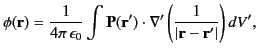(201)

where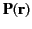is the electric polarization (i.e., the electric dipole moment per unit volume), and the integral is over all space.Next: Charge Sheets and Dipole Up: Electrostatic Fields Previous: Electrostatic Energy
Richard Fitzpatrick 2014-06-27